# AP Statistics : How to interpret dotplots

## Example Questions

### Example Question #1 : How To Interpret Dotplots

A basketball coach wants to determine if a player's height can be used to predict the number of points that player scores in a season.  Before using a statistical test to determine the precise relationship of the variables, the coach wants a visual of the data to see if there is likely to be a relationship.  Which of the following should the coach create?

Scatterplot

Histogram

Bar chart

Z-score

Bell curve

Scatterplot

Explanation:

A scatterplot is a diagram that shows the values of two variables and provides a general illustration of the relationship between them.

### Example Question #1 : Using Scatter Plots

Based on the scatter plot below, is there a correlation between theandvariables? If so, describe the correlation.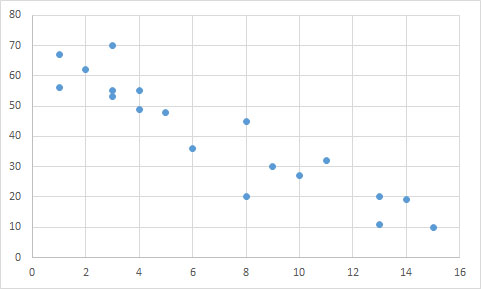Yes; negative linear relationship

Yes; positive linear relationship

Yes; negative exponential relationship

No; there is no correlation

Yes; negative linear relationship

Explanation:

The data points follow an overall linear trend, as opposed to being randomly distributed. Though there are a few outliers, there is a general relationship between the two variables.

A line could accurately predict the trend of the data points, suggesting there is a linear correlation. Since the y-values decrease as the x-values increase, the correlation must be negative. We can see that a line connecting the upper-most and lower-most points would have a negative slope.

An exponential relationship would be curved, rather than straight.

### Example Question #2 : How To Interpret Dotplots

Order the correlation coefficients to fit the order of the following graphs (two coefficients will not be used),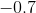,,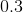,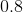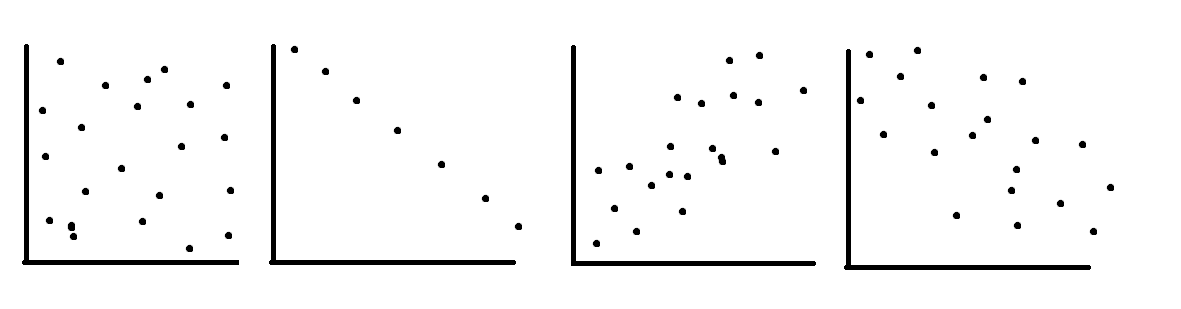,,,,,,,,,,,,The first graph is random scatter, no correlation, the second is perfect linear, corellation, the last two have fairly strong positive and negative corellations, the student should know that a corellation ofis much weaker than them